# 312320.pptx

Mar. 25, 2023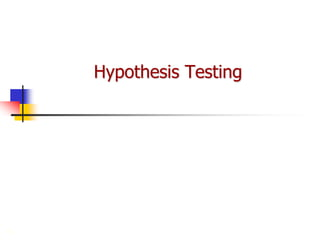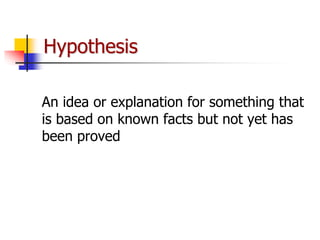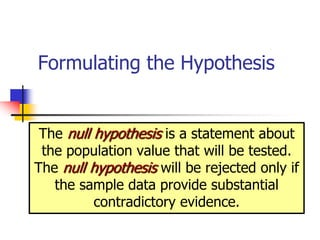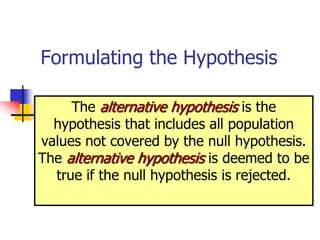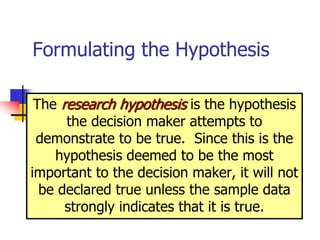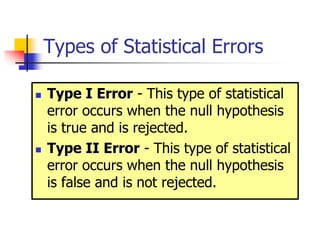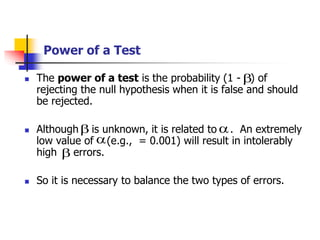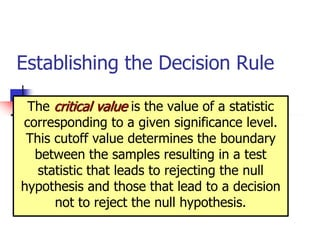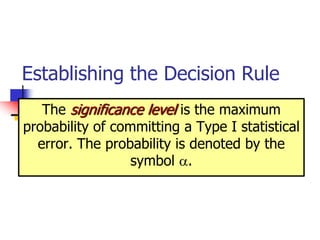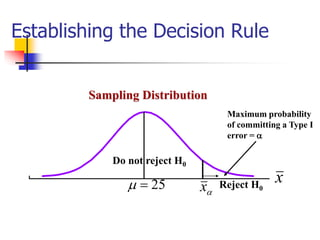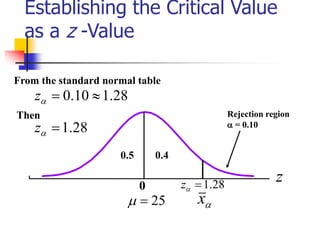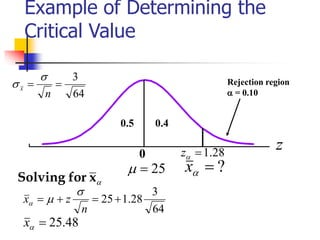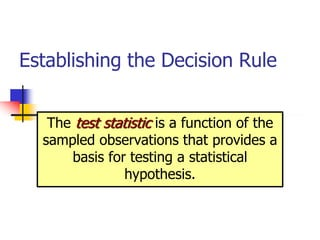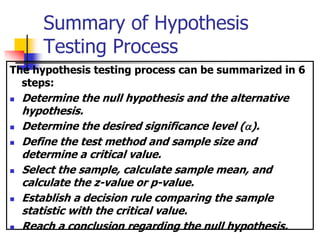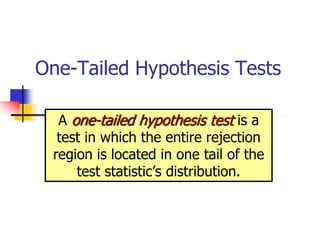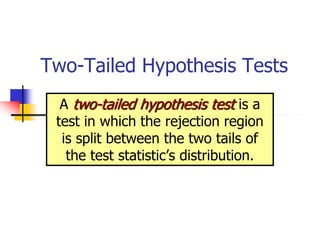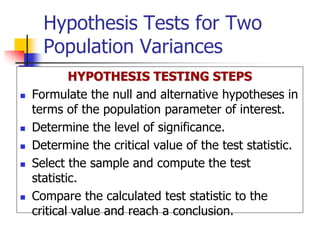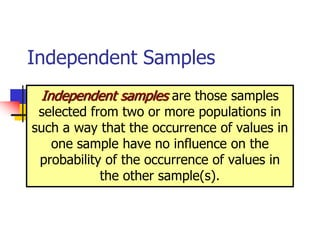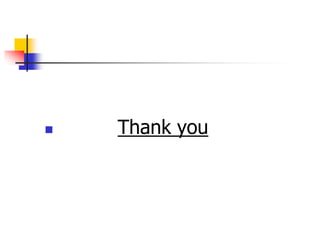1 of 19

### 312320.pptx

2. Hypothesis An idea or explanation for something that is based on known facts but not yet has been proved
3. Formulating the Hypothesis The null hypothesis is a statement about the population value that will be tested. The null hypothesis will be rejected only if the sample data provide substantial contradictory evidence.
4. Formulating the Hypothesis The alternative hypothesis is the hypothesis that includes all population values not covered by the null hypothesis. The alternative hypothesis is deemed to be true if the null hypothesis is rejected.
5. Formulating the Hypothesis The research hypothesis is the hypothesis the decision maker attempts to demonstrate to be true. Since this is the hypothesis deemed to be the most important to the decision maker, it will not be declared true unless the sample data strongly indicates that it is true.
6. Types of Statistical Errors  Type I Error - This type of statistical error occurs when the null hypothesis is true and is rejected.  Type II Error - This type of statistical error occurs when the null hypothesis is false and is not rejected.
7.  The power of a test is the probability (1 - ) of rejecting the null hypothesis when it is false and should be rejected.  Although is unknown, it is related to . An extremely low value of (e.g., = 0.001) will result in intolerably high errors.  So it is necessary to balance the two types of errors. Power of a Test          
8. Establishing the Decision Rule The critical value is the value of a statistic corresponding to a given significance level. This cutoff value determines the boundary between the samples resulting in a test statistic that leads to rejecting the null hypothesis and those that lead to a decision not to reject the null hypothesis.
9. Establishing the Decision Rule The significance level is the maximum probability of committing a Type I statistical error. The probability is denoted by the symbol .
10. Reject H0  x x 25   Do not reject H0 Sampling Distribution Maximum probability of committing a Type I error =  Establishing the Decision Rule
11.  x z 25   Rejection region  = 0.10 28 . 1   z 0 From the standard normal table 28 . 1 10 . 0    z Then 28 . 1   z 0.5 0.4 Establishing the Critical Value as a z -Value
12. ?   x z 25   Rejection region  = 0.10 28 . 1   z 0 0.5 0.4 Example of Determining the Critical Value 64 3   n x    x for Solving 64 3 28 . 1 25    n z x    48 . 25   x
13. Establishing the Decision Rule The test statistic is a function of the sampled observations that provides a basis for testing a statistical hypothesis.
14. Summary of Hypothesis Testing Process The hypothesis testing process can be summarized in 6 steps:  Determine the null hypothesis and the alternative hypothesis.  Determine the desired significance level ().  Define the test method and sample size and determine a critical value.  Select the sample, calculate sample mean, and calculate the z-value or p-value.  Establish a decision rule comparing the sample statistic with the critical value.  Reach a conclusion regarding the null hypothesis.
15. One-Tailed Hypothesis Tests A one-tailed hypothesis test is a test in which the entire rejection region is located in one tail of the test statistic’s distribution.
16. Two-Tailed Hypothesis Tests A two-tailed hypothesis test is a test in which the rejection region is split between the two tails of the test statistic’s distribution.
17. Hypothesis Tests for Two Population Variances HYPOTHESIS TESTING STEPS  Formulate the null and alternative hypotheses in terms of the population parameter of interest.  Determine the level of significance.  Determine the critical value of the test statistic.  Select the sample and compute the test statistic.  Compare the calculated test statistic to the critical value and reach a conclusion.
18. Independent Samples Independent samples are those samples selected from two or more populations in such a way that the occurrence of values in one sample have no influence on the probability of the occurrence of values in the other sample(s).
19.  Thank you